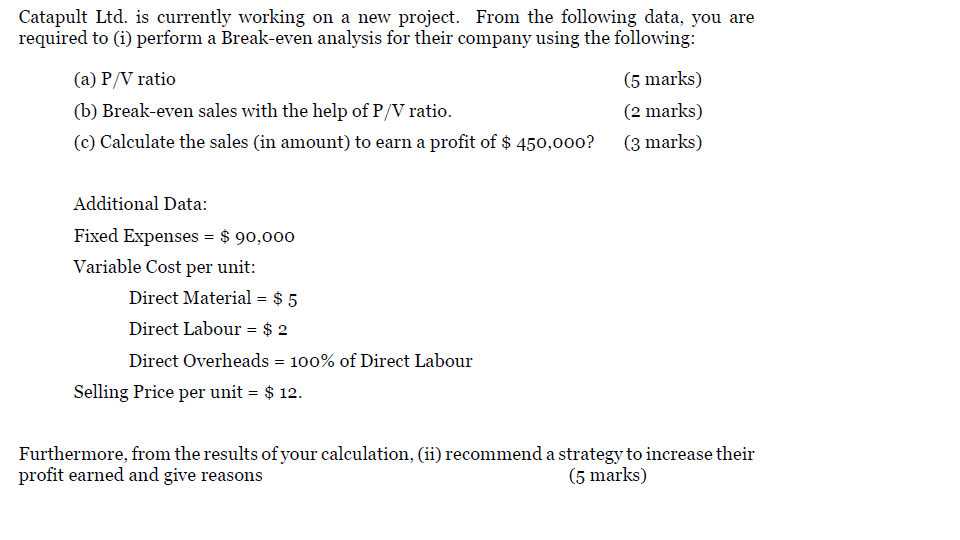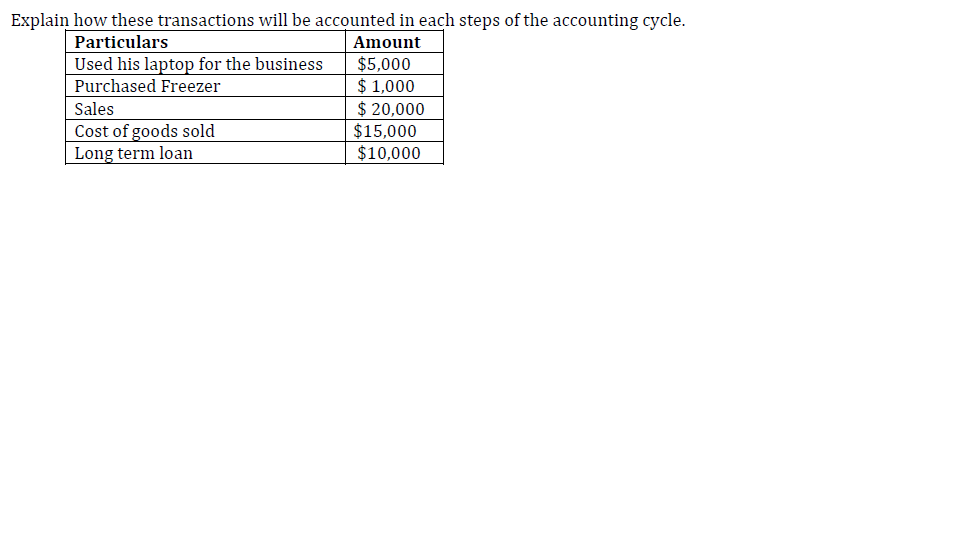# Question & Answer: Catapult Ltd. is currently working on a new project. From the following data, you are required to (i) perform a Brea…..

Please answer BOTH questions for THUMBS UP, ELSE SOLUTION WILL NOT BE RATED.

Ques 1

Don't use plagiarized sources. Get Your Custom Essay on
Question & Answer: Catapult Ltd. is currently working on a new project. From the following data, you are required to (i) perform a Brea…..
GET AN ESSAY WRITTEN FOR YOU FROM AS LOW AS \$13/PAGEQues 2Catapult Ltd. is currently working on a new project. From the following data, you are required to (i) perform a Break-even analysis for their company using the following: (5 marks) ( marks) (c) Calculate the sales (in amount) to earn a profit of \$ 450,ooo? (3 marks) (a) P/V ratio (b) Break-even sales with the help of P/V ratio Additional Data: Fixed Expenses \$9o,ooo Variable Cost per unit: Direct Material = \$ 5 Direct Labour-2 Direct Overheads 100% of Direct Labour Selling Price per unit\$12. Furthermore, from the results ofyour calculation, (ii) recommend a strategy to increase their profit earned and give reasons (5 marks)

Solution:

First of all please note as per Chegg policy if two separate questions are asked in a question and both questions are different from each other, the experts may answer both question but it is not mandatory to answer both the question. Hence answer for first question is as follows:

1)

(i)

(a) PV Ratio = Contribution Margin / Selling Price x 100

Contribution Margin = Selling Price – Variable Cost = Selling price \$12 – (Direct material 5 + Direct labor 2 + Variable Overhead 2) = 12 – 9 = 3

PV Ratio = Contribution Margin 3 / Selling Price 12 x 100 = 25%

(b) Break Even Sales = Total Fixed Cost / PV ratio = 90,000 / 25% or 0.25 = \$360,000

(c) Sales to earn a profit of \$450,000 = (Total Fixed Cost + Desired Profit) / PV Ratio

= (90,000 + 450,000) / 25%

= \$2,160,000

(ii) Strategy to increase their profit

Profit is the difference of Sales Revenue and total expenses. Hence in order to increase the profit, we need to look on Selling Price and total expenses.

We can increase the profit

• To reduce the direct material cost
• To reduce the direct labor cost